# RS Aggarwal Solutions for Class 8 Maths Chapter 16 - Parallelograms

RS Aggarwal Solutions For Class 8 Maths Chapter 16 Parallelograms are provided here. You can download the pdf of RS Aggarwal Solutions for Class 8 Maths Chapter 16 Parallelograms from the given links. RS Aggarwal Solutions helps students to attain a good score in the examinations, while also providing extensive knowledge about the subject, as Class 8 is a critical stage in their academic career. Therefore, we at BYJU’S provide answers to all questions uniquely and briefly.

RS Aggarwal Solutions For Class 8 Maths also provides the foundation to higher studies. RS Aggarwal Solutions For Class 8 Maths Chapter 16 Parallelograms provides the concepts in an effective way.

## Download pdf of RS Aggarwal Solutions For Class 8 Maths Chapter 16 Parallelograms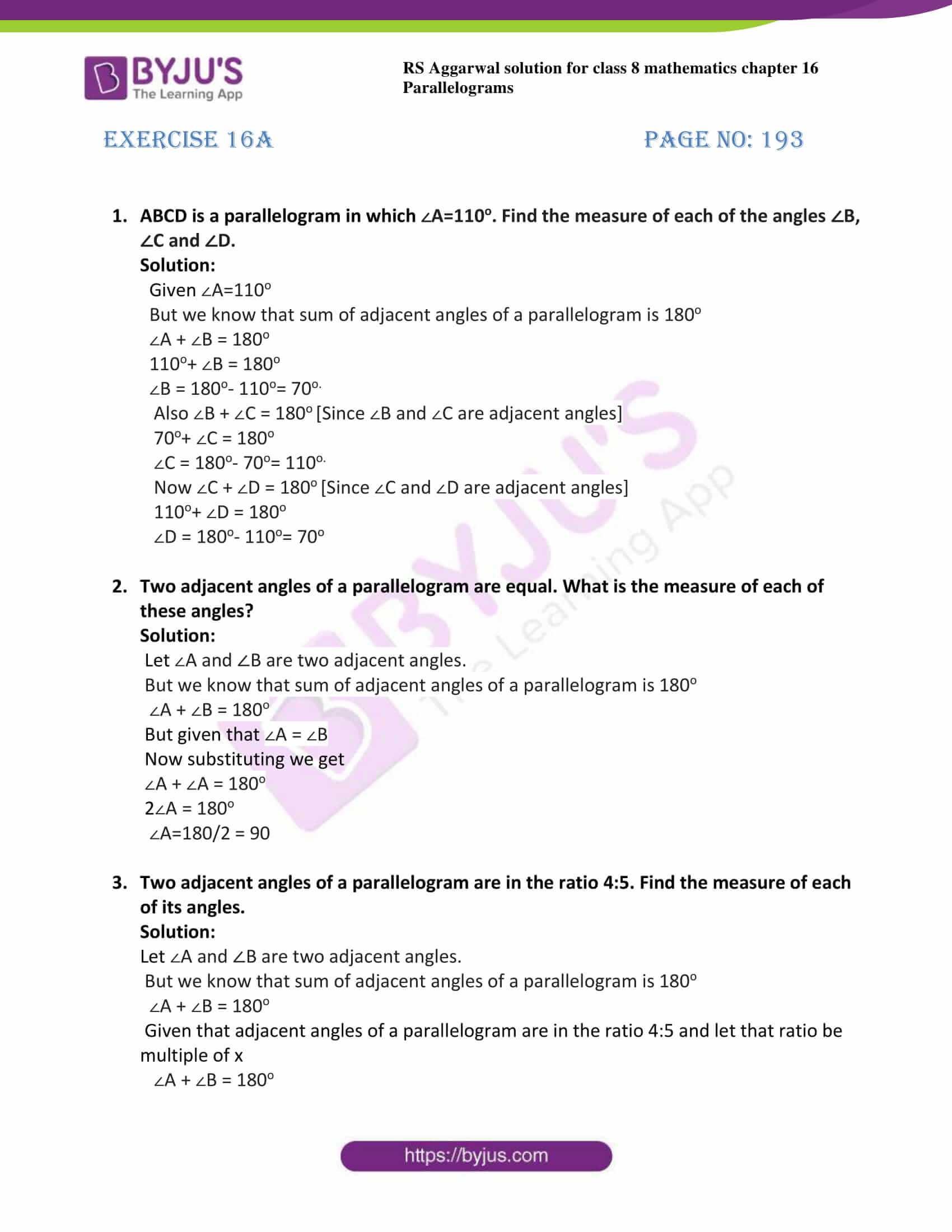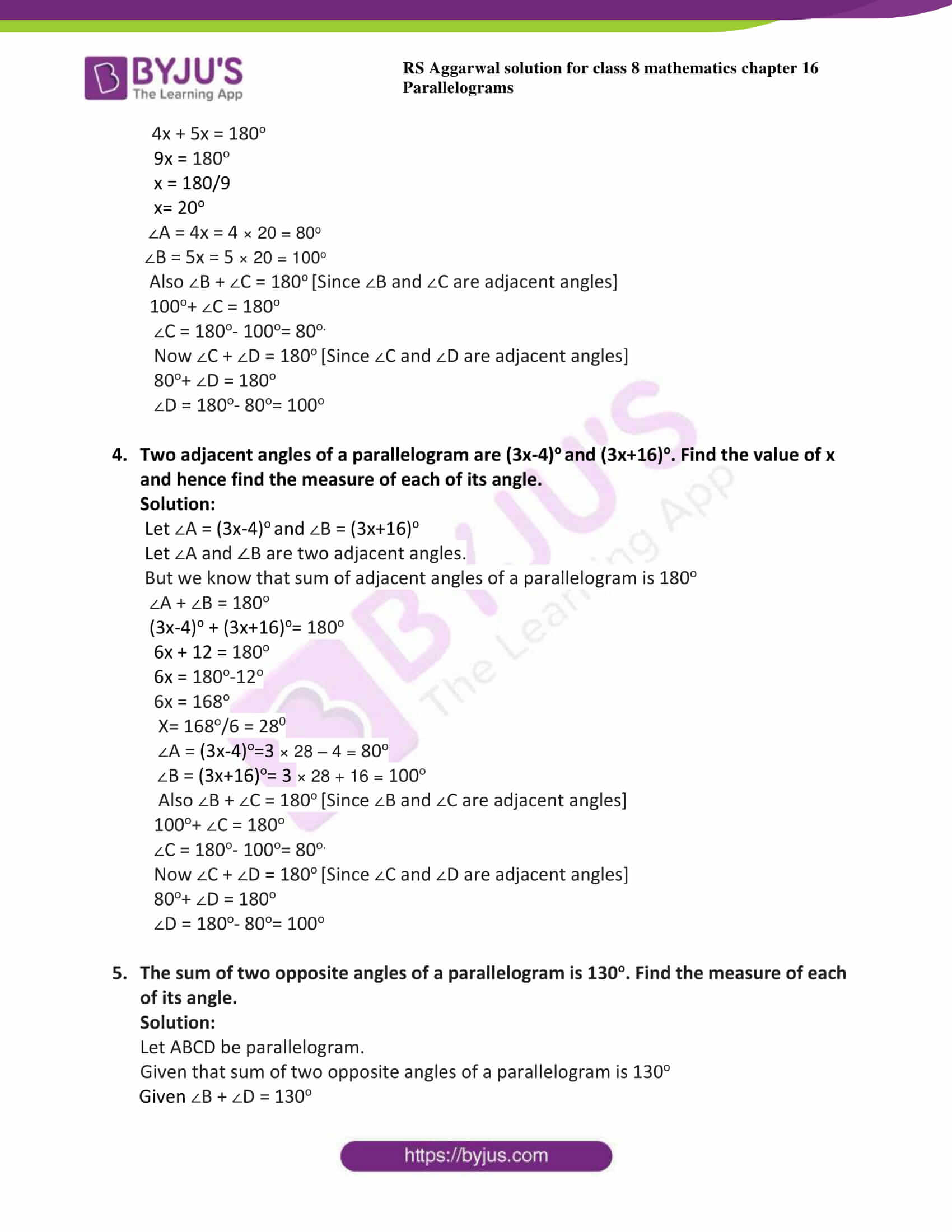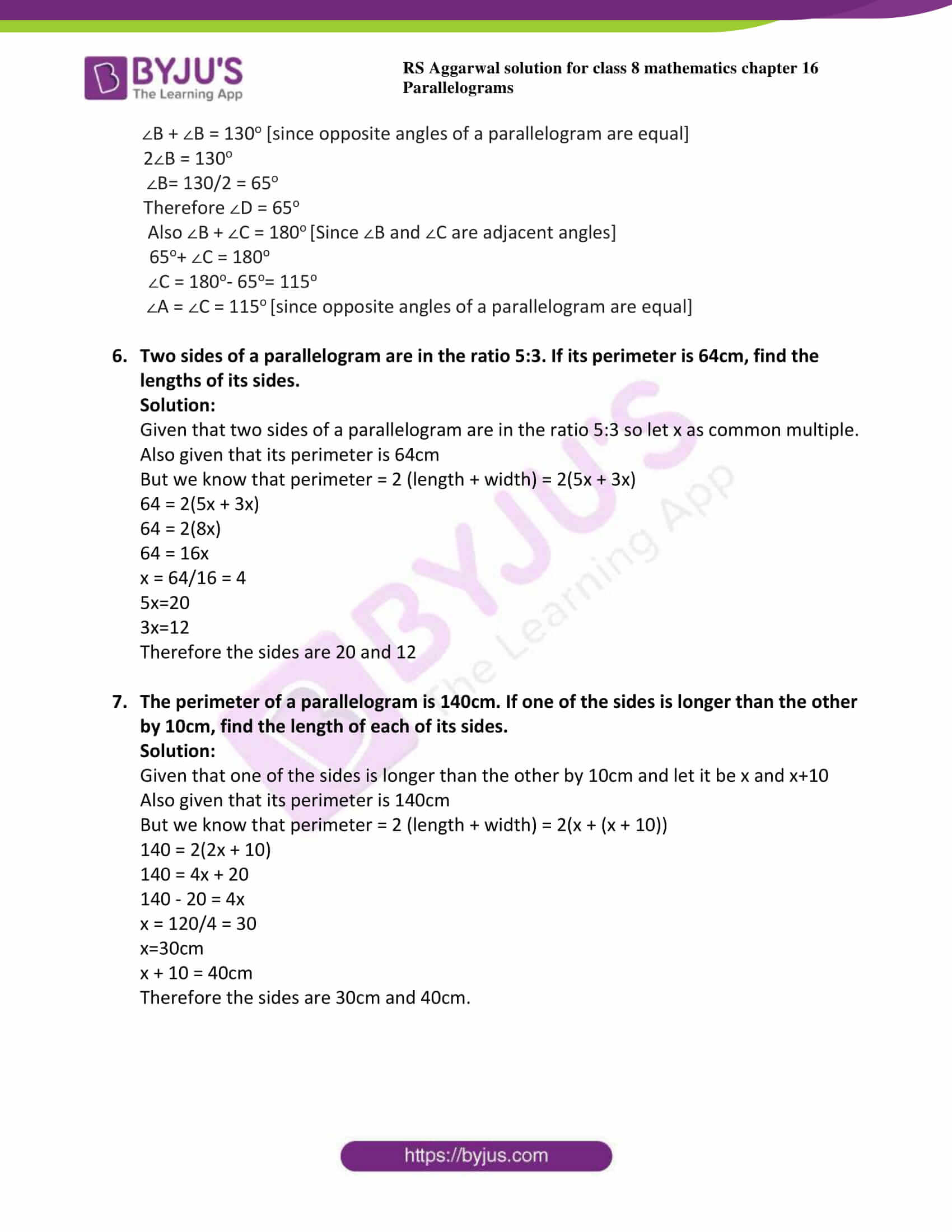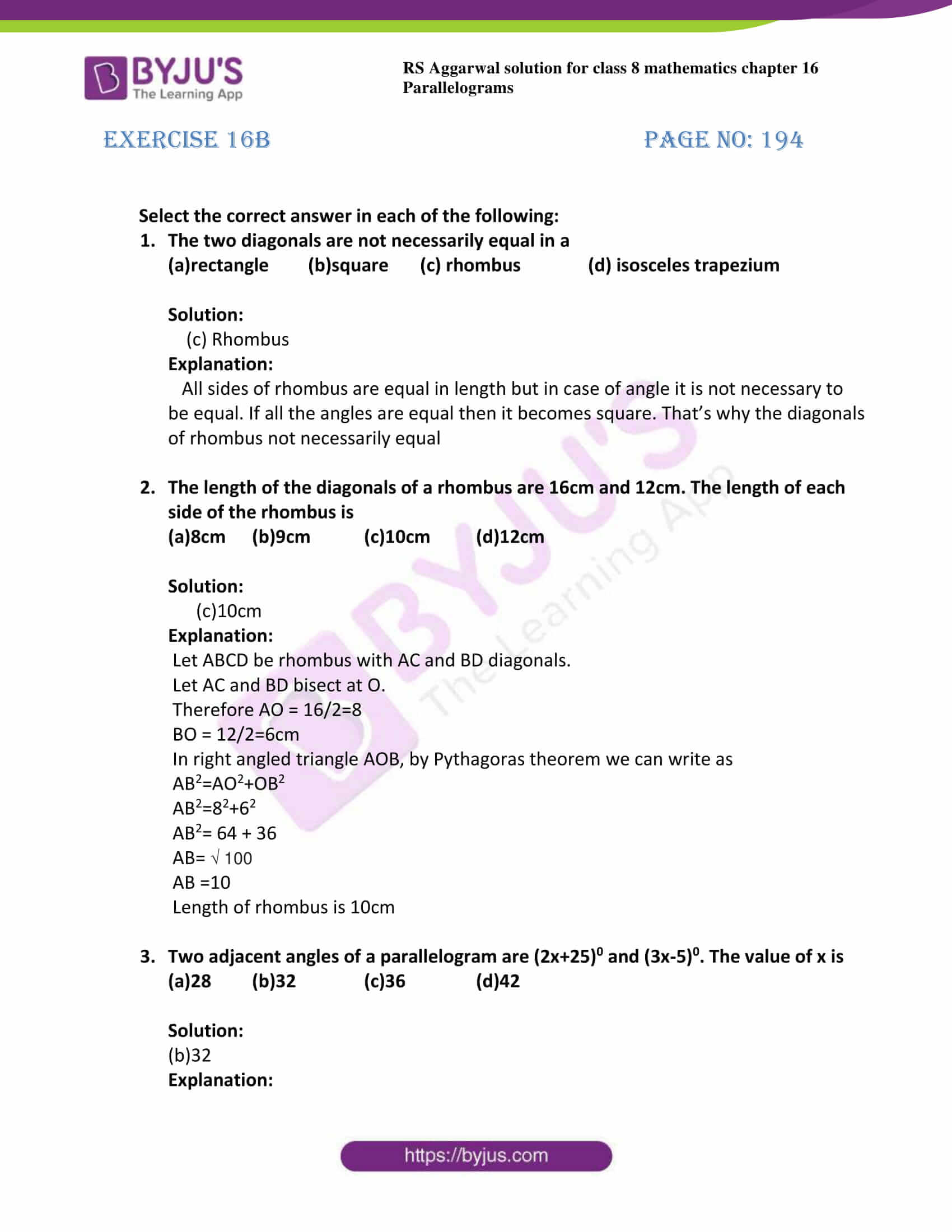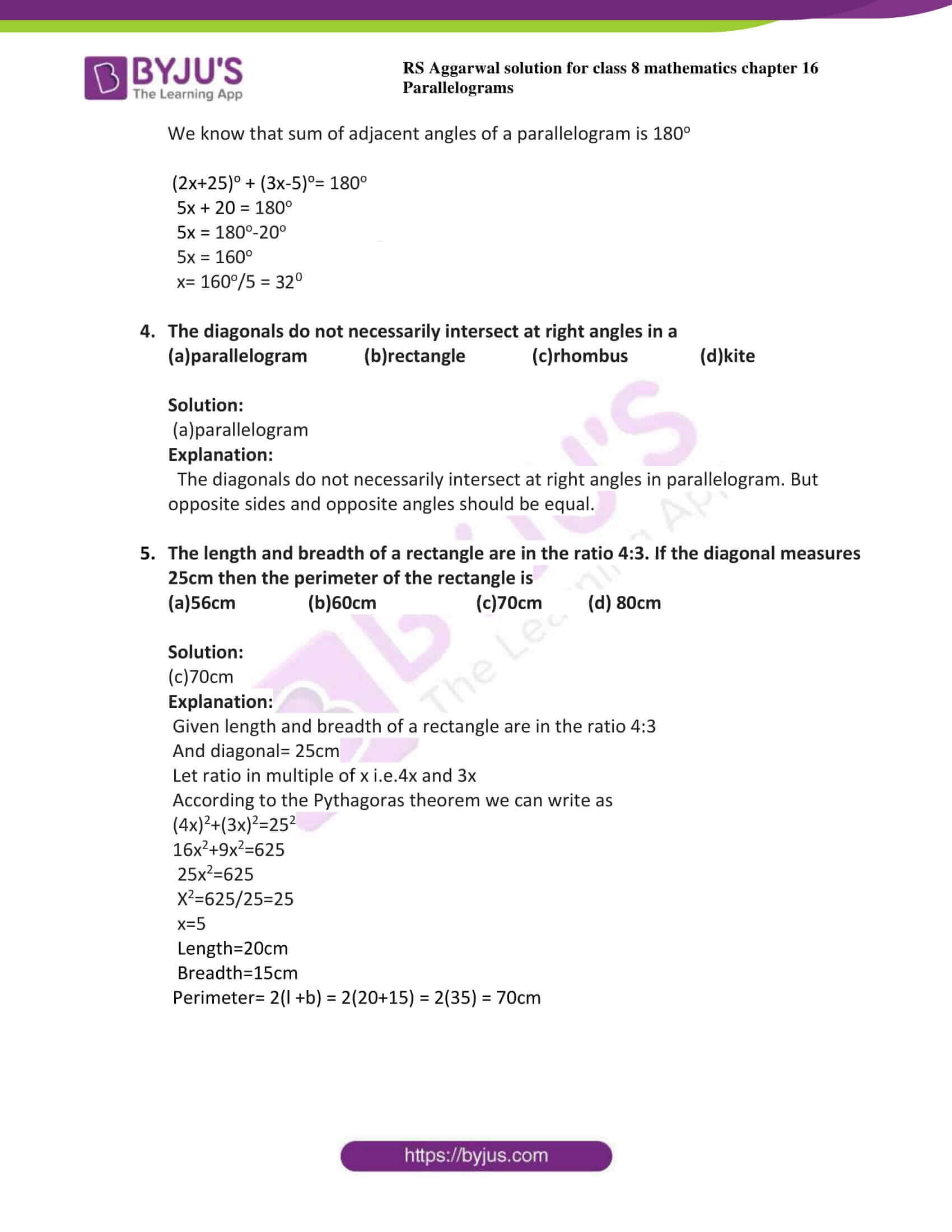## Exercise 16A Page No: 193

1. ABCD is a parallelogram in which ∠A=110o. Find the measure of each of the angles ∠B, ∠C and ∠D.

Solution:

Given ∠A=110o

But we know that sum of adjacent angles of a parallelogram is 180o

∠A + ∠B = 180o

110o+ ∠B = 180o

∠B = 180o– 110o= 70o.

Also ∠B + ∠C = 180o [Since ∠B and ∠C are adjacent angles]

70o+ ∠C = 180o

∠C = 180o– 70o= 110o.

Now ∠C + ∠D = 180o [Since ∠C and ∠D are adjacent angles]

110o+ ∠D = 180o

∠D = 180o– 110o= 70o

2. Two adjacent angles of a parallelogram are equal. What is the measure of each of these angles?

Solution:

Let ∠A and ∠B are two adjacent angles.

But we know that sum of adjacent angles of a parallelogram is 180o

∠A + ∠B = 180o

But given that ∠A = ∠B

Now substituting we get

∠A + ∠A = 180o

2∠A = 180o

∠A=180/2 = 90

3. Two adjacent angles of a parallelogram are in the ratio 4:5. Find the measure of each of its angles.

Solution:

Let ∠A and ∠B are two adjacent angles.

But we know that sum of adjacent angles of a parallelogram is 180o

∠A + ∠B = 180o

Given that adjacent angles of a parallelogram are in the ratio 4:5 and let that ratio be multiple of x

∠A + ∠B = 180o

4x + 5x = 180o

9x = 180o

x = 180/9

x= 20o

∠A = 4x = 4 × 20 = 80o

∠B = 5x = 5 × 20 = 100o

Also ∠B + ∠C = 180o [Since ∠B and ∠C are adjacent angles]

100o+ ∠C = 180o

∠C = 180o– 100o= 80o.

Now ∠C + ∠D = 180o [Since ∠C and ∠D are adjacent angles]

80o+ ∠D = 180o

∠D = 180o– 80o= 100o

4. Two adjacent angles of a parallelogram are (3x-4)o and (3x+16)o. Find the value of x and hence find the measure of each of its angle.

Solution:

Let ∠A = (3x-4)o and ∠B = (3x+16)o

Let ∠A and ∠B are two adjacent angles.

But we know that sum of adjacent angles of a parallelogram is 180o

∠A + ∠B = 180o

(3x-4)o + (3x+16)o= 180o

6x + 12 = 180o

6x = 180o-12o

6x = 168o

X= 168o/6 = 280

∠A = (3x-4)o=3 × 28 – 4 = 80o

∠B = (3x+16)o= 3 × 28 + 16 = 100o

Also ∠B + ∠C = 180o [Since ∠B and ∠C are adjacent angles]

100o+ ∠C = 180o

∠C = 180o– 100o= 80o.

Now ∠C + ∠D = 180o [Since ∠C and ∠D are adjacent angles]

80o+ ∠D = 180o

∠D = 180o– 80o= 100o

5. The sum of two opposite angles of a parallelogram is 130o. Find the measure of each of its angle.

Solution:

Let ABCD be parallelogram.

Given that sum of two opposite angles of a parallelogram is 130o

Given ∠B + ∠D = 130o

∠B + ∠B = 130o [since opposite angles of a parallelogram are equal]

2∠B = 130o

∠B= 130/2 = 65o

Therefore ∠D = 65o

Also ∠B + ∠C = 180o [Since ∠B and ∠C are adjacent angles]

65o+ ∠C = 180o

∠C = 180o– 65o= 115o

∠A = ∠C = 115o [since opposite angles of a parallelogram are equal]

6. Two sides of a parallelogram are in the ratio 5:3. If its perimeter is 64cm, find the lengths of its sides.

Solution:

Given that two sides of a parallelogram are in the ratio 5:3 so let x as common multiple.

Also given that its perimeter is 64cm

But we know that perimeter = 2 (length + width) = 2(5x + 3x)

64 = 2(5x + 3x)

64 = 2(8x)

64 = 16x

x = 64/16 = 4

5x=20

3x=12

Therefore the sides are 20 and 12

7. The perimeter of a parallelogram is 140cm. If one of the sides is longer than the other by 10cm, find the length of each of its sides.

Solution:

Given that one of the sides is longer than the other by 10cm and let it be x and x+10

Also given that its perimeter is 140cm

But we know that perimeter = 2 (length + width) = 2(x + (x + 10))

140 = 2(2x + 10)

140 = 4x + 20

140 – 20 = 4x

x = 120/4 = 30

x=30cm

x + 10 = 40cm

Therefore the sides are 30cm and 40cm.

## Exercise 16B

Select the correct answer in each of the following:

1. The two diagonals are not necessarily equal in a

(a)rectangle (b)square (c) rhombus (d) isosceles trapezium

Solution:

(c) Rhombus

Explanation:

All sides of rhombus are equal in length but in case of angle it is not necessary to be equal. If all the angles are equal then it becomes square. That’s why the diagonals of rhombus not necessarily equal

2. The length of the diagonals of a rhombus are 16cm and 12cm. The length of each side of the rhombus is

(a)8cm (b)9cm (c)10cm (d)12cm

Solution:

(c)10cm

Explanation:

Let ABCD be rhombus with AC and BD diagonals.

Let AC and BD bisect at O.

Therefore AO = 16/2=8

BO = 12/2=6cm

In right angled triangle AOB, by Pythagoras theorem we can write as

AB2=AO2+OB2

AB2=82+62

AB2= 64 + 36

AB= √ 100

AB =10

Length of rhombus is 10cm

3. Two adjacent angles of a parallelogram are (2x+25)0 and (3x-5)0. The value of x is

(a)28 (b)32 (c)36 (d)42

Solution:

(b)32

Explanation:

We know that sum of adjacent angles of a parallelogram is 180o

(2x+25)o + (3x-5)o= 180o

5x + 20 = 180o

5x = 180o-20o

5x = 160o

x= 160o/5 = 320

4. The diagonals do not necessarily intersect at right angles in a

(a)parallelogram (b)rectangle (c)rhombus (d)kite

Solution:

(a)parallelogram

Explanation:

The diagonals do not necessarily intersect at right angles in parallelogram. But opposite sides and opposite angles should be equal.

5. The length and breadth of a rectangle are in the ratio 4:3. If the diagonal measures 25cm then the perimeter of the rectangle is

(a)56cm (b)60cm (c)70cm (d) 80cm

Solution:

(c)70cm

Explanation:

Given length and breadth of a rectangle are in the ratio 4:3

And diagonal= 25cm

Let ratio in multiple of x i.e.4x and 3x

According to the Pythagoras theorem we can write as

(4x)2+(3x)2=252

16x2+9x2=625

25x2=625

X2=625/25=25

x=5

Length=20cm

Perimeter= 2(l +b) = 2(20+15) = 2(35) = 70cm

## RS Aggarwal Solutions for Class 8 Maths Chapter 16- Parallelograms

Chapter 16, Parallelograms, contains 2 Exercises. RS Aggarwal Solutions given here contains the answers to all the questions present in these exercises. Let us have a look at some of the concepts that are being discussed in this chapter.

• Definition of parallelogram
• Definition of rhombus
• Definition of rectangle
• Definition of square
• Definition of trapezium
• Definition of isosceles trapezium
• Properties of parallelogram
• Diagonal properties of a rectangle
• Diagonal properties of a rhombus
• Diagonal properties of a square

Exercise 16A

Exercise 16B

### Chapter Brief of RS Aggarwal Solutions for Class 8 Maths Chapter 16 – Parallelograms

The RS Aggarwal Solutions for Class 8 Maths Chapter 15 – Parallelograms deals with the definition of parallelogram, definition of some geometrical shapes like rhombus, rectangle, square, trapezium, isosceles trapezium. It also deals with properties of parallelograms, diagonal properties of a rectangle, diagonal properties of rhombus and diagonal properties of a square.